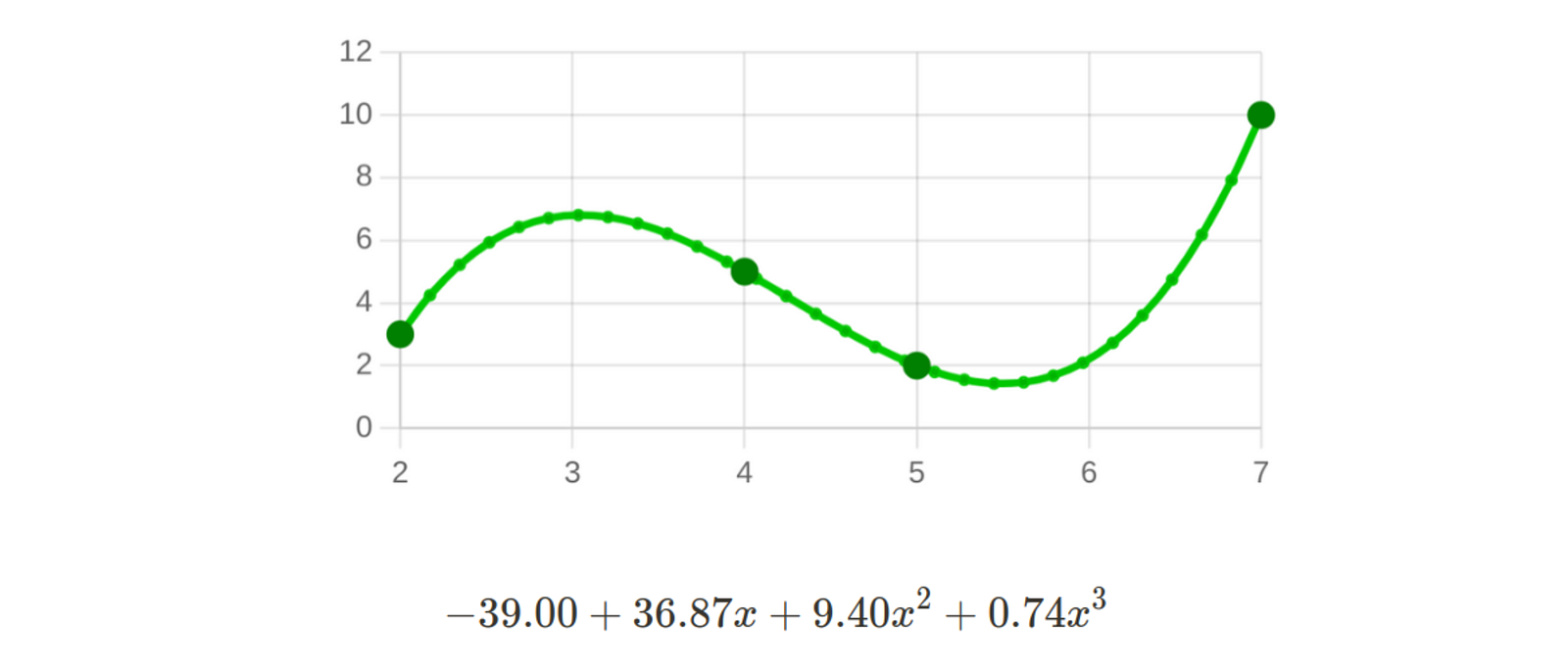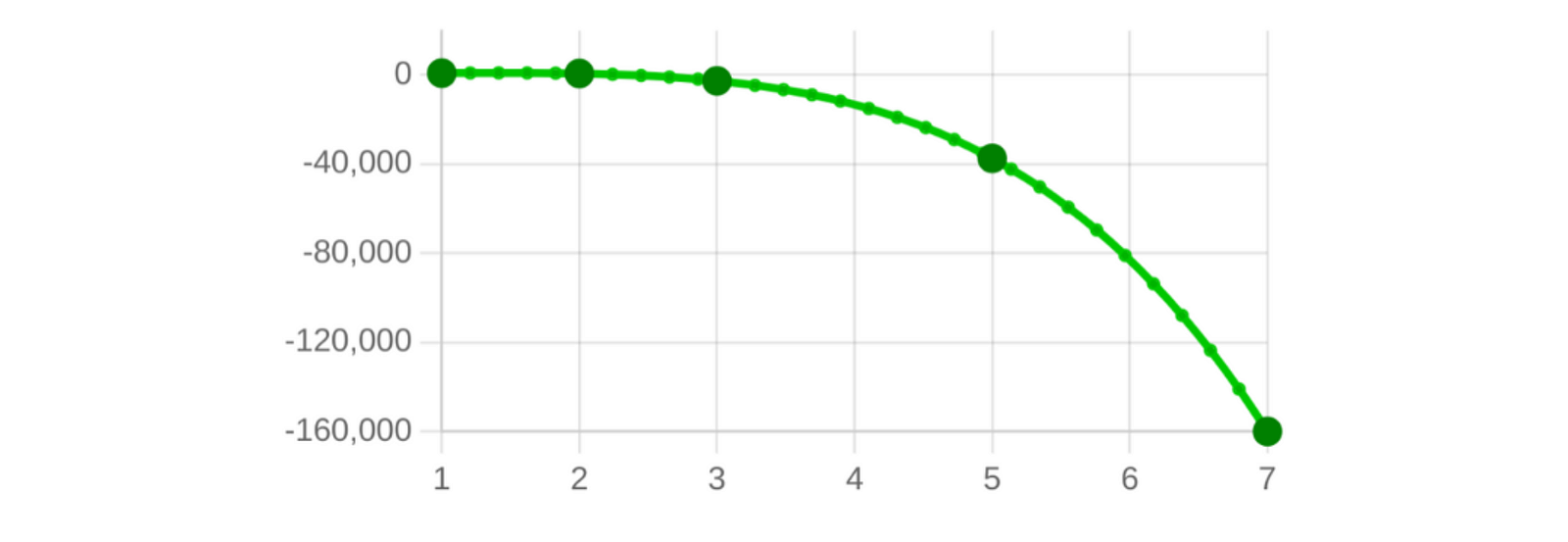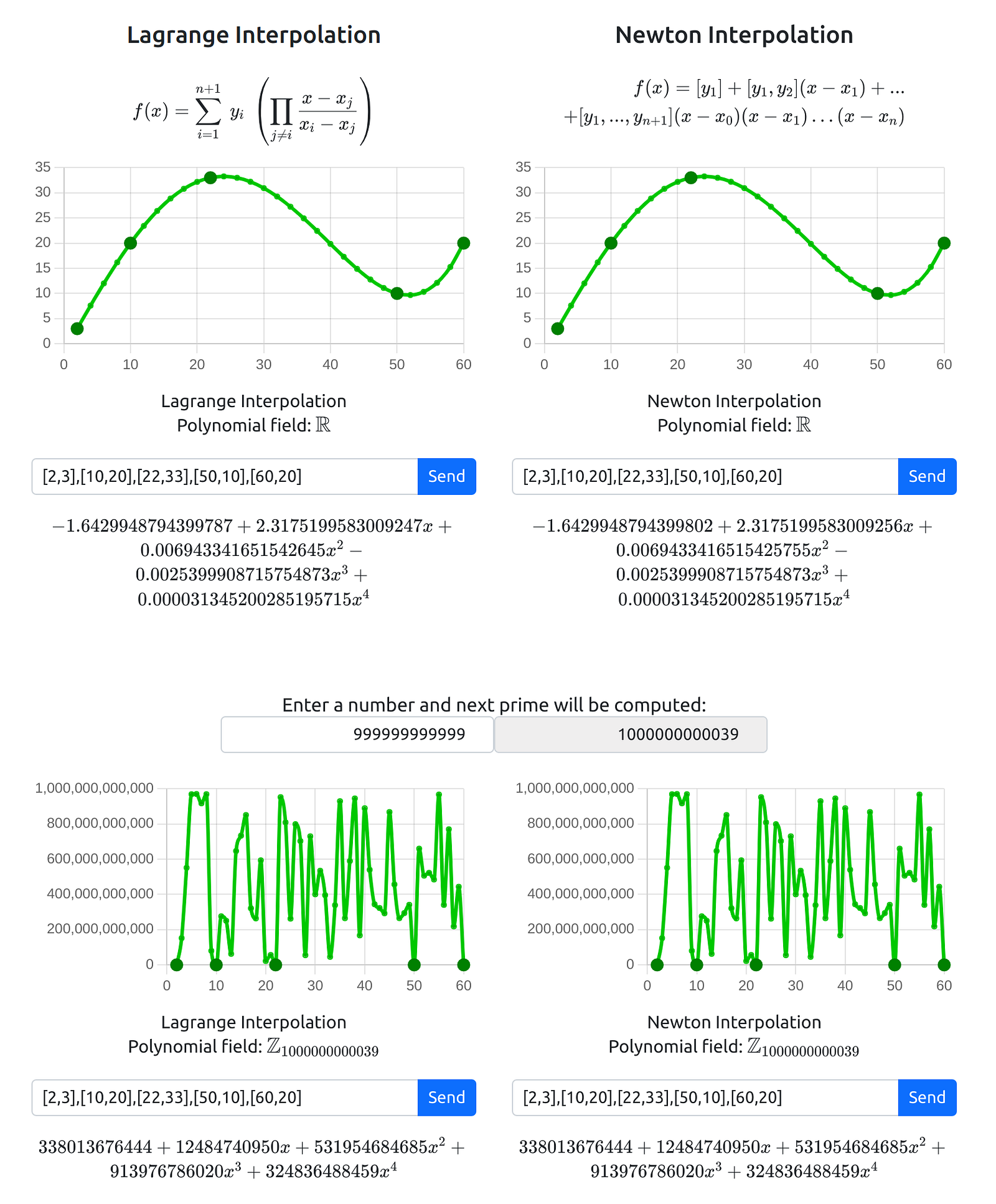# Sharing a Secret With Polynomial Interpolation

Featured on HashnodeI have always believed that programming and mathematics go well together, even though I'm not an expert in either area, I enjoy learning about them. I usually visit Hacker News, where I sometimes find very interesting articles. Recently, I came across a link that led me to the book “A Programmer’s Introduction to Mathematics” by Jeremy Kun, an excellent book that uses programming to help readers understand and integrate mathematical concepts through practical examples.

The first chapter talks about polynomials. Despite studying algebra in university, I had never approached this topic the way the book presents it. I was unfamiliar with polynomial interpolation and how it can be used in cryptography.

Jeremy explains how to share a secret number using polynomial interpolation, based on the original idea presented in the paper “How To Share a Secret” by Adi Shamir.

One of the exercises in the book is to create a web app that uses this mechanism so that users can generate a secret number and share it with specific people. When I came across this exercise, it seemed completely unattainable to me. I had taken courses on Node, React, databases, algorithms, data structures… (by the way, I highly recommend Zero To Mastery Academy) but I had never faced the challenge of building a web app completely on my own. This exercise pushed me to do it and also allowed me to apply mathematical knowledge that I didn’t even fully understand. Below, I will explain the process and the code I used.

### Mathematical foundation

Polynomials have a property that says the following:

For any integer $$n \geq 0$$ and any list of $$n+1$$ points $$(x_1, y_1), (x_2, y_2), ..., (x_{n+1}, y_{n+1})$$ in $$\mathbb{R}^2$$ with $$x_1 < x_2 < ... < x_{n+1}$$, there exists a unique polynomial $$p(x)$$ of degree at most $$n$$ such that $$p(x_i)=y_i$$for all $$i$$

For example, if we have the following 4 points: [2,3], [4,5], [5,2], [7,10], there is a unique polynomial degree 4 that passes through those points.There are several ways to obtain this polynomial, with the most well-known methods being Lagrange’s method and Netwon’s method.

The point is that, as described by Adi Shamir, this property can be used to securely share a secret among multiple people. Let’s say we have 5 friends and we want to share a secret with them, represented as a string interpreted as an integer. The problem is that if I simply give them the secret, one of them could do something malicious. We don’t want to give them a part of the security code, they could try to force the rest and gain access. What I need is a scheme that has the following properties:

1. Each friend has a “share”, which means a unique number for them

2. If 4 of the friends collude without the 5th code, they cannot reconstruct the secret.

3. If all 5 friends combine their shares, then they can reconstruct the secret.

Polynomial interpolation allows us to achieve this! Additionally, there is no restriction on the number of people we want to share the secret with, and we can also configure it so that it doesn’t require everyone to agree, but perhaps only a smaller group.

Let’s see this with an example. Let’s imagine we want to generate a secret number and share it with 7 people, but it should only require 5 people to agree to discover it. Here’s the procedure.

• We generate an integer number, let’s call it s, which will be the secret. For example, let’s say $$s=129$$.

• We generate a random polynomial of degree 4 (since 5 people are needed to discover it), such that $$f(0)=s$$. For example:

$$f(x) = 129 + 931x-201x^2+103x^3-80x^4$$

• We distribute the shares to the 7 people, in this case, they would be as follows:

$$(1,f(1)), (2,f(2)), (3,f(3)), (4,f(4)), (5,f(5)), (6,f(6)), (7,f(7))$$

For the example polynomial given, the shares would be as follows:

 Share id Share Value 1 882 2 731 3 -2586 4 -13251 5 -37366 6 -82953 7 -159954

In this way, the only way to obtain the secret (129) is for 5 people to agree and share their shares. By finding the polynomial that passes through these 5 points, they can evaluate $$f(0)$$ and gain access to the secret.

For example, if we have the following points: [1,882], [3,-2586], [5,-37366], [7,-159954], [2,731], we will obtain the polynomial from the graph, which is the one we generated previously.This is the mechanism! I find it simple and powerful. Let’s consider that we are talking about storing a secret number, but practically any type of information can be encoded numerically.

# Web-app

Creating the web app was a beautiful challenge, as I mentioned before. I had knowledge of Node, React, and programming in general, but I had never done something entirely on my own. It was a long process where I learned a lot along the way. It’s absolutely true that when you try to put theory into practice, that’s when problems arise. And in trying to solve them, that’s when you truly integrate your knowledge. You can find the Github repository on this link.

I started by creating an API with Node, preparing certain endpoints. For example, I created an endpoint that received an array of points and returned the corresponding polynomial using Lagrange interpolation. Lagrange interpolation is based on constructing the following polynomial:

$$f(x)=\sum_{i=1}^{n+1} \ y_i \ \left( \prod_{j \neq i} \frac{x-x_j}{x_i-x_j} \right)$$

This is the function that generates the polynomial:

const lagrangeInterpolationFieldReal = (points) => {
let baseSum = new Polynomial(, "float");

for (let i = 0; i < points.length; i++) {
let baseProd = new Polynomial(, "float");

for (let j = 0; j < points.length; j++) {
if (i !== j) {
let term = new Polynomial([-points[j], 1], "float");
term = term.mul(
new Polynomial([1 / (points[i] - points[j])], "float")
);
baseProd = baseProd.mul(term);
}
}
baseProd.mul(new Polynomial([points[i]], "float"))
);
}

return baseSum;
};


As explained by Adi Shamir in his article, and also Jeremy Kun, the best way to generate these secrets and polynomials is by working with modular arithmetic instead of real numbers. When I first read about this, I didn’t understand anything at all.

When it is mentioned to “work in the field mod(p),” it refers to performing operations and calculations using modular arithmetic. This involves working with numbers in a finite set, particularly the field of integers modulo p.

The mod(p) field is a set of integers ranging from 0 to (p-1), where p is a prime number. In this field, mathematical operations are performed modulo p, which means that if the result of an operation exceeds p, the remainder of the division by p is taken. For example, in mod(7), if you perform the operation 5+4, the result is 2, since 9 divided by 7 has a remainder of 2.

Working with modular arithmetic is very beneficial for our secret-sharing mechanism because we are only working with integers, eliminating fractions and approximations.

Furthermore, as mentioned by Kun, modular arithmetic is faster for arbitrarily large integers. When evaluating f(x) at an unknown value that is not in mod(p), the size of the result and the knowledge of the degree of f can provide information about the value of the input x. In the case of sharing secrets, its size reveals information about the coefficients of the polynomial, which is problematic if we aim for complete confidentiality.

Here I present the modified version of the function that performs Lagrange interpolation using modular arithmetic:

const lagrangeInterpolationFieldModP = (points, prime) => {
points = points.map((point) => [BigInt(point), BigInt(point)]);
prime = BigInt(prime);

let baseSum = new Polynomial([0n], "bigint");

for (let i = 0; i < points.length; i++) {
let baseProd = new Polynomial([1n], "bigint");

for (let j = 0; j < points.length; j++) {
if (i !== j) {
let term = new Polynomial([-points[j] % prime, 1n], "bigint");
term = term.mul(
new Polynomial(
[
modDivide(
1n,
((points[i] % prime) - (points[j] % prime)) % prime,
BigInt(prime)
),
],
"bigint"
)
);
term = term.mod(prime);
baseProd = baseProd.mul(term);
baseProd = baseProd.mod(prime);
}
}
baseProd.mul(new Polynomial([points[i] % prime], "bigint")).mod(prime)
);
baseSum = baseSum.mod(prime);
}

return baseSum;
};

const modDivide = (numerator, denominator, p) => {
const denominatorModP = ((denominator % p) + p) % p;
const inverse = modInverse(denominatorModP, p);
const result = (numerator * inverse) % p;
return (result + p) % p;
};

const modInverse = (num, p) => {
const [gcd, x, y] = extendedEuclidean(num, p);
if (gcd !== 1n) {
throw new Error("The multiplicative inverse does not exist in modulo p.");
}
const result = ((x % p) + p) % p;
return result;
};

const extendedEuclidean = (a, b) => {
if (b === 0n) {
return [a, 1n, 0n];
}
const [gcd, x1, y1] = extendedEuclidean(b, a % b);
const x = y1;
const y = x1 - ((a / b) | 0n) * y1;
return [gcd, x, y];
};


Once the initial endpoints of the API were completed, I tried to design a GUI as simple as possible. I thought of having 3 buttons:

• Generate Secret:
Allows the user to create a secret by specifying the number of people they want to share it with and the number of shares required to reconstruct it. Once this information is provided, the user can download an Excel file containing the shares to distribute them.

• Decode Secret:
Allows the user to upload an Excel file with the necessary shares to uncover the secret. If the secret is successfully decoded, a window will open displaying the graph of the polynomial and the value of the secret, which is $$f(0)$$.

• Clear Secret:
Allows the user to delete the currently stored secret.

Initially, I did all of this for a single user, and the database was a simple JSON file. However, the challenge arose to make it multi-user with authentication and allow each user to save their secret in a database. I had done something similar in the Zero To Mastery Node course, so I decided to venture into it.

The solution I came up with is probably highly improvable, but it works. I used authentication with Google OAuth 2.0 and a database with MongoDB Atlas.

The web app was already finished, and I had solved the exercise that Kun proposed in his book! I couldn’t believe it myself. Afterward, I thought it would be a good idea to provide an “Interpolation Playground” where users could “play” with polynomial interpolation, both in the field of real numbers and in modular arithmetic. I also wanted to demonstrate that both the Lagrange and Newton methods produce the same polynomial.

Among other things, I found it important to include this section so that the user can truly understand the benefits of modular arithmetic and how the size of the secret depends on the size of the chosen prime number. For example:

• List of points: [2, 3], [10, 20], [22, 33], [50, 10], [60, 20]

• Prime number: 1000000000039As can be seen, Lagrange and Newton interpolation produce the same polynomial. However, when working with real numbers, the numbers have decimals and approximations that make them not exactly equal. Additionally, the coefficients of the polynomial have very different sizes. On the other hand, when using modular arithmetic, both solutions are identical, and the coefficients of the polynomials are always between 0 and p (the chosen prime number).